GFG App
Open AppBrowser
Continue

# Find maximum values & position in columns and rows of a Dataframe in Pandas

In this article, we are going to discuss how to find the maximum value and its index position in columns and rows of a Dataframe.

## Python3

 `import` `numpy as np` `import` `pandas as pd` `# List of Tuples` `matrix ``=` `[(``10``, ``56``, ``17``),` `          ``(np.NaN, ``23``, ``11``),` `          ``(``49``, ``36``, ``55``),` `          ``(``75``, np.NaN, ``34``),` `          ``(``89``, ``21``, ``44``)` `          ``]` `# Create a DataFrame` `abc ``=` `pd.DataFrame(matrix, index``=``list``(``'abcde'``), columns``=``list``(``'xyz'``))`   `# output` `abc`

Output: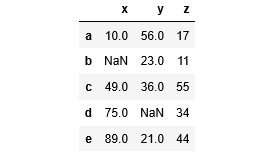Time complexity: O(n) where n is the number of elements in the matrix.
Auxiliary space: O(n) where n is the number of elements in the matrix.

## Find maximum values in columns and rows in Pandas

Pandas dataframe.max() method finds the maximum of the values in the object and returns it. If the input is a series, the method will return a scalar which will be the maximum of the values in the series. If the input is a Dataframe, then the method will return a series with a maximum of values over the specified axis in the Dataframe. The index axis is the default axis taken by this method.

### Get the maximum values of every column in Python

To find the maximum value of each column, call the max() method on the Dataframe object without taking any argument. In the output, We can see that it returned a series of maximum values where the index is the column name and values are the maxima from each column.

## Python3

 `# find the maximum of each column` `maxValues ``=` `abc.``max``()`   `print``(maxValues)`

Output: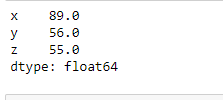### Get max value from a row of a Dataframe in Python

For the maximum value of each row, call the max() method on the Dataframe object with an argument axis=1. In the output, we can see that it returned a series of maximum values where the index is the row name and values are the maxima from each row.

## Python3

 `# find the maximum values of each row` `maxValues ``=` `abc.``max``(axis``=``1``)` `print``(maxValues)`

Output: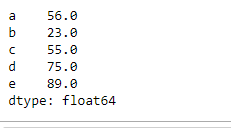### Get the maximum values of every column without skipping NaN in Python

From the above examples, NaN values are skipped while finding the maximum values on any axis. By putting skipna=False we can include NaN values also. If any NaN value exists it will be considered as the maximum value.

## Python3

 `# find maximum value of each` `# column without skipping NaN` `maxValues ``=` `abc.``max``(skipna``=``False``)`   `print``(maxValues)`

Output: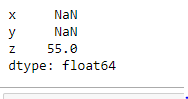### Get maximum values from multiple columns in Python

To get the maximum value of a single column see the following example

## Python3

 `# find maximum value of a` `# single column 'x'` `maxClm ``=` `df[``'x'``].``max``()`   `# Another method is:` `# maxClm = df.max()['x']`   `print``(``"Maximum value in column 'x': "``)` `print``(maxClm)`

Output: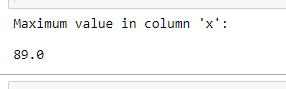### Get max value in one or more columns

A list of columns can also be passed instead of a single column to find the maximum values of specified columns

## Python3

 `# find maximum values of a list of columns` `maxValues ``=` `df[[``'x'``, ``'z'``]].``max``()`   `print``(``"Maximum value in column 'x' & 'z': "``)` `print``(maxValues)`

Output: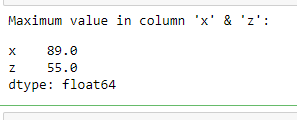## Find the maximum position in columns and rows in Pandas

Pandas dataframe.idxmax() method returns the index of the first occurrence of maximum over the requested axis. While finding the index of the maximum value across any index, all NA/null values are excluded.

### Find the row index which has the maximum value

It returns a series containing the column names as index and row as index labels where the maximum value exists in that column.

## Python3

 `# find the index position of maximum` `# values in every column` `maxValueIndex ``=` `df.idxmax()`   `print``(``"Maximum values of columns are at row index position :"``)` `print``(maxValueIndex)`

Output: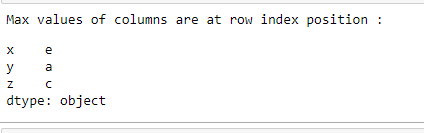### Find the column name which has the maximum value

It returns a series containing the rows index labels as index and column names as values where the maximum value exists in that row.

## Python3

 `# find the column name of maximum` `# values in every row` `maxValueIndex ``=` `df.idxmax(axis``=``1``)`   `print``(``"Max values of row are at following columns :"``)` `print``(maxValueIndex)`

Output: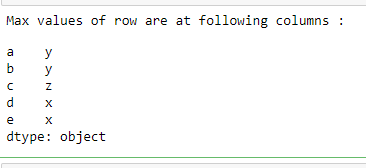My Personal Notes arrow_drop_up